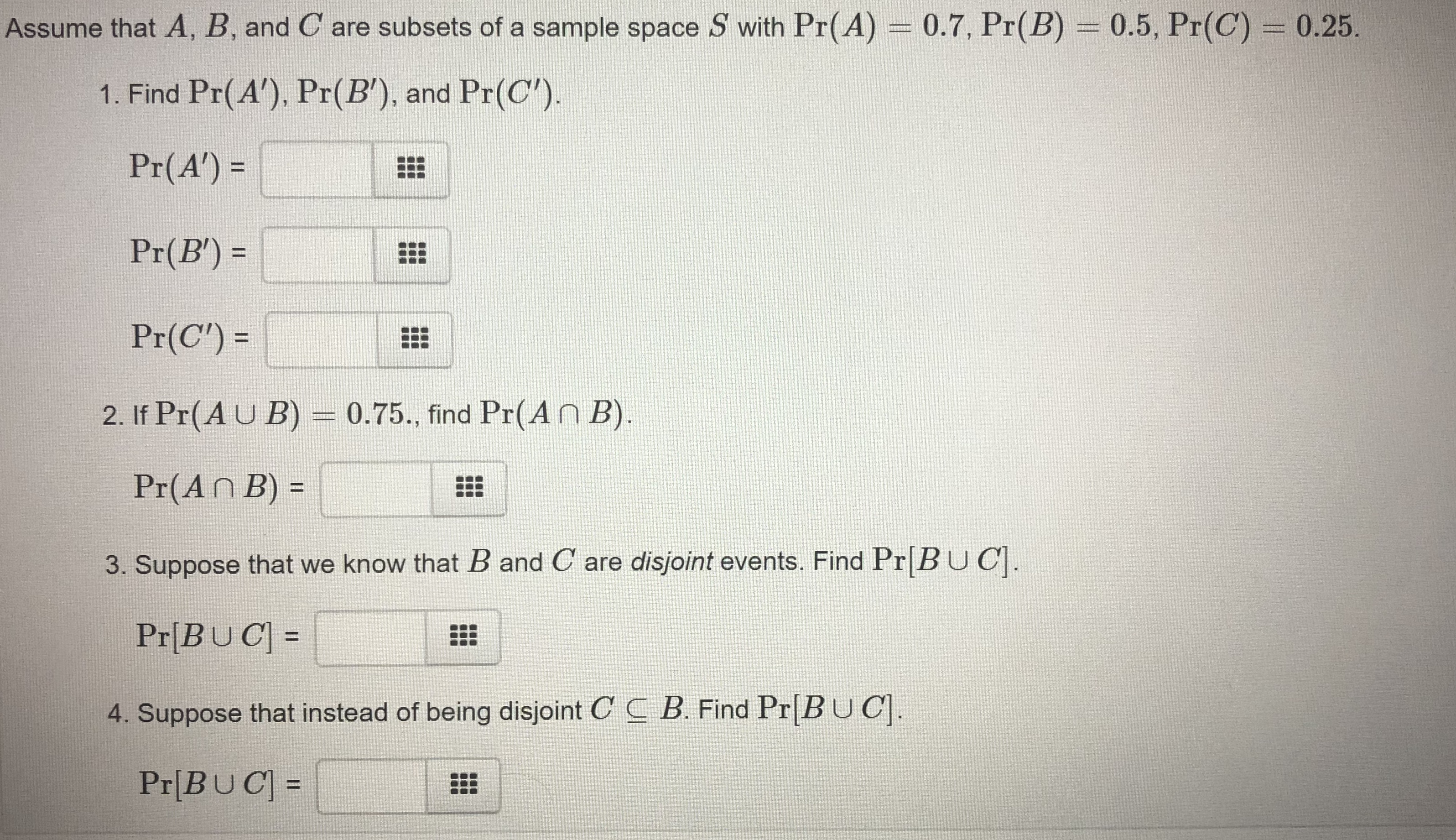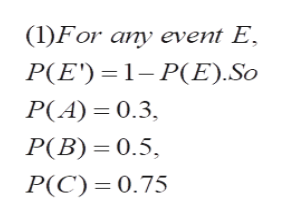Assume that A, B, and C are subsets of a sample space S with Pr(A) = 0.7, Pr(B) = 0.5, Pr(C) = 0.25.1. Find Pr(A). Pr(B'), and Pr(C').Pr(A')Pr(B)Pr(C')=2. If Pr(AU B) = 0.75., find Pr(An B).Pr(An B) =3. Suppose that we know that B and C are disjoint events. Find Pr|BUC.Pr[BUC4. Suppose that instead of being disjoint C C B. Find Pr BUCPr[BUC =

Questionhelp_outlineImage TranscriptioncloseAssume that A, B, and C are subsets of a sample space S with Pr(A) = 0.7, Pr(B) = 0.5, Pr(C) = 0.25. 1. Find Pr(A). Pr(B'), and Pr(C'). Pr(A') Pr(B) Pr(C')= 2. If Pr(AU B) = 0.75., find Pr(An B). Pr(An B) = 3. Suppose that we know that B and C are disjoint events. Find Pr|BUC. Pr[BUC 4. Suppose that instead of being disjoint C C B. Find Pr BUC Pr[BUC = fullscreen
Step 1

To compute the required probabilities

Step 2

As per norms , the first three questions are answered.

Step 3help_outlineImage Transcriptionclose(1)For any event E P(E) P(E).So P(A) 0.3 P(B) 0.5 P(C) 0.75 fullscreen

Want to see the full answer?

See Solution

Want to see this answer and more?

Our solutions are written by experts, many with advanced degrees, and available 24/7

See Solution
Tagged in

Math